Browse by Topic
Related Topics

# 2 Pi or Not 2 Pi?

Three months ago the world (or at least the geek world) celebrated Pi Day of the Century (3/14/15…). Today (6/28) is another math day: 2π-day, or Tau Day (2π = 6.28319…).

Some say that Tau Day is really the day to celebrate, and that τ(=2π) should be the most prominent constant, not π. It all started in 2001 with the famous opening line of a watershed essay by Bob Palais, a mathematician at the University of Utah:

“I know it will be called blasphemy by some, but I believe that π is wrong.”

Which has given rise in some circles to the celebration of Tau Day—or, as many people say, the one day on which you are allowed to eat two pies.

But is it true that τ is the better constant? In today’s world, it’s quite easy to test, and the Wolfram Language makes this task much simpler. (Indeed, Michael Trott’s recent blog post on dates in pi—itself inspired by Stephen Wolfram’s Pi Day of the Century post—made much use of the Wolfram Language.) I started by looking at 320,000 preprints from arXiv.org to see in practice how many formulas involve 2π rather than π alone, or other multiples of π.

Here is a WordCloud of some formulas containing 2π: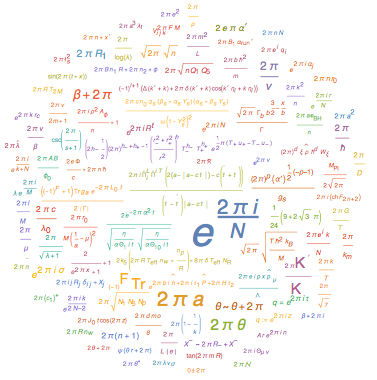I found that only 18% of formulas considered involve 2π, suggesting that τ, after all, would not be a better choice.

But then why do τ supporters believe that we should switch to this new symbol? One reason is that using τ would make geometry and trigonometry easier to understand and learn. After all, when we learn trigonometry, we don’t measure angles in degrees, but in radians, and there are 2π radians in a circle. This means that 1/4 of a circle corresponds to 1/2 π radians, or π/2, and not a quarter of something! This counterintuitive madness would be resolved by the symbol τ, because every ratio of the circle would have a matching ratio of τ. For example, 1/4 would have an angle of τ/4.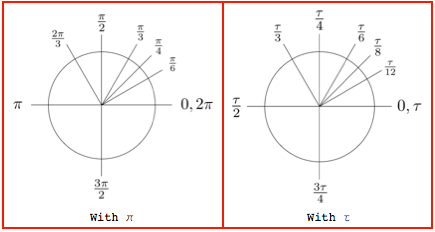I personally do not have strong feelings against π, and to be honest, I don’t think students would learn trigonometry faster if they were to use τ. Think about the two most important trigonometric functions, sine and cosine. What’s most helpful to remember about them is that sin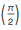= cos(2 π) = 1, and sin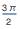= cos(π) = –1. I have not only always preferred cosine simply because it’s easier to remember (there are no fractions in π and 2 π), I’ve also always recognized that sine and cosine are different because one is nonzero on integer multiples of π and the other is nonzero on some fractions of it. By using τ instead, this symmetry would be lost, and we would be left with the equalities sin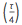= cos(τ) = 1 and sin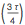= cos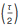= –1.

Given these observations, it seems like choosing τ or π is a personal choice. That’s fair, but it’s not a rigorous approach for determining which constant is more useful.

Even the approach I had at the beginning could lead to the wrong conclusion. The Tau Manifesto, by Michael Hartl, gives some examples of places where 2π is most commonly used: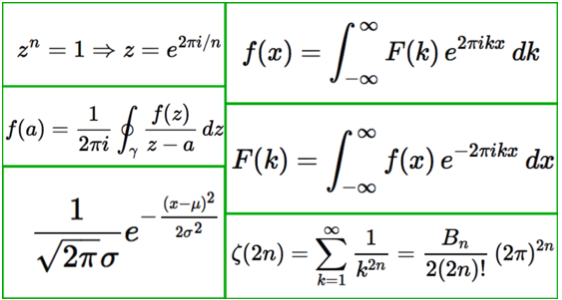And indeed, all these formulas would be easier if we used τ. However, those are just six of the vast number of formulas that scientists use regularly, and as I mentioned before, not many mathematical expressions involve 2π. Nevertheless, it could happen that formulas not involving 2π would still be simpler if written in τ. For example, the expression 4 π² would simply become (τ²).

For this reason I looked back at the scientific articles to see whether using τ instead of 2π (and τ/2 instead of π) would make their formulas simpler. For instance, these are some that would be simpler in τ: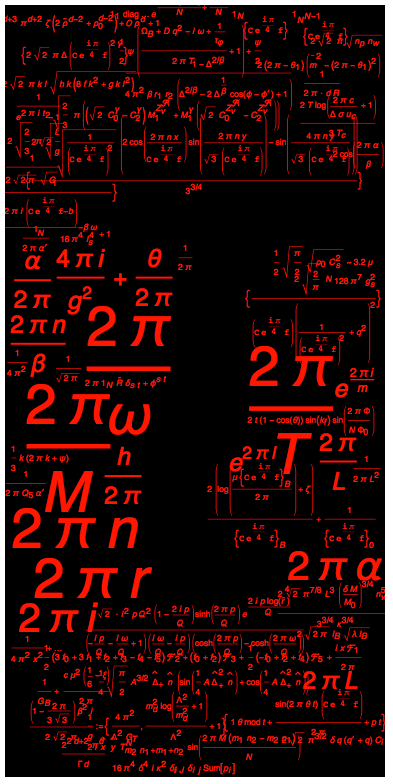And these are some that would not: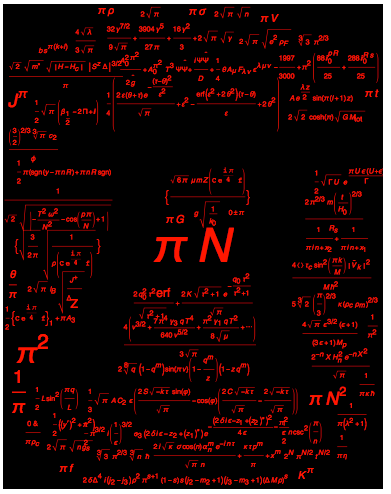Let me now try to explain what I mean by simpler by looking at an example: if I take the term containing π in the bottom-left formula of the Tau Manifesto equation table: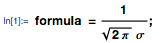I can replace π with τ/2 using ReplaceAll, and I get: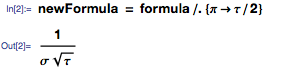Just by looking at these two expressions, you can see that the second one is simpler. It’s not just your intuition that tells you that; it’s clear that there are fewer symbols and constants in the replaced expression. We can look at their corresponding TreeForms to demonstrate it explicitly: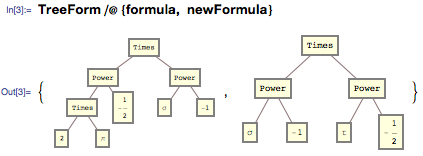To get a numeric difference, we can look at the leaf counts (number of leaves on the trees), which correspond to the number of symbols and constants in the original formulas: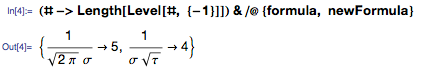To see whether τ had an overall simplifying impact, I computed the complexity of each formula (defined as their leaf counts, as computed above) involving π that appeared in the articles when using π and τ. To be more precise, I first deleted all the formulas that were either equal to π or 2 π. I felt it would have been unfair to consider those as well because very often, if they appear by themselves, they do not stand for formulas. I then compared the number of times the τ formulas were better with the number of times they were not, and only 43% of the formulas whose complexity changed at all were actually better, meaning that using τ would make more than half of them look more complex. In other words, based on this comparison, we should keep using π. However, this is not the end of the story.

One observation I made is that if an expression gets either more or less complex, it’s likely to have a leaf count that is less than 40. In fact, if you look at the percentage of formulas that are better when using π or τ and that have a number of leaves that is less than a fixed number, you get this picture: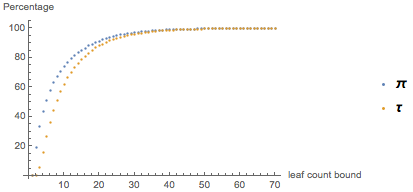where the x axis represents the upper bound on the number of leaves. This suggests that almost all formulas that become simpler have complexities less than 50, regardless of the symbol we choose.

A more relevant observation is that the situation changes drastically as the complexity of the formulas increases. Already by only considering formulas that have complexities greater than 3, like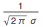from earlier, only 48% are simpler in π against 52% that are simpler in τ. The graph below shows how the percentage of formulas that are better in either π or τ changes as a function of the complexity: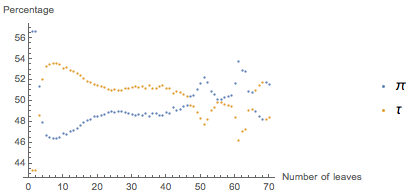As you can see, as the number of leaves exceeds 48, the situation becomes chaotic. This is because only 0.4% of formulas have complexities greater than 50. There are not enough of these for us to deduce anything stable and reasonable about them, and the previous observation tells us that we should not really worry much about them anyway.

What this graph tells me is that in everyday life, and for anything more complex than fairly easy expressions like, we should indeed use τ for simplicity. But there is still something else I have not considered. What about different subjects?

It might be that formulas in physics look simpler in τ, but formulas in other subjects do not. The initial search I made included articles from different subjects; however, I didn’t initially check whether the majority of π-containing formulas were from a limited subset of those subjects, or whether the ones that became simpler with τ were mostly from a limited subset. In fact, if I just restrict analysis to articles in mathematics, the situation becomes the following: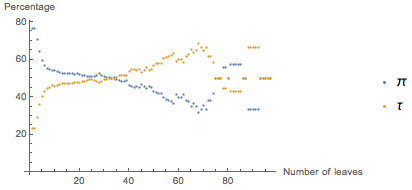Basically, only 23% of formulas benefit from using τ, and those benefits come only when the complexity is fairly high. For instance, something of this sort: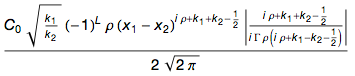would be an expression that would be simpler in τ, and you probably have not seen many of this type of expression. This suggests that either scientists in different subjects should use different conventions depending on their field-specific formulas, or that all scientific disciplines should switch to τ even though it does not really make sense for some of them to do so. After all, in a democracy, the majority wins, and it is impossible to accommodate everyone.

However, the above formula shows something else that I want to point out. With τ, it becomes this: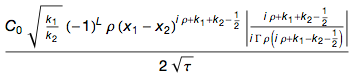And that is not much of an improvement: even though an expression could be easier in τ, the improvement might be so small that it is irrelevant. Consider for instance these two expressions together with their leaf counts: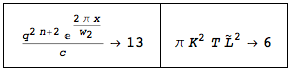And the corresponding expressions in τ: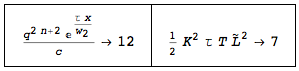The first formula is simpler in τ, but the leaf count is only 1/13 smaller than the original complexity, whereas the second expression is simpler in π and the replaced expression is 1/6 higher than the original complexity. In other words, the first case’s improvement was 1/13 and the second’s was -1/6 (the minus sign indicates negative improvement, as the expression in τ was worse). The mean of the vector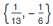is –0.044, a negative number, which means that using τ in these two expressions makes the whole vector 0.044 worse, although π and τ each improved one formula.

This vector approach is different from the one-count-per-equation one that I used earlier. It considers quantity of improvement instead of just an either/or binary, and it completely reverses the previous conclusions. I have computed these vectors for formulas having complexities bounded from below in the same way I did in the previous example. What I’ve seen is that the overall improvement in going from π to τ, computed as the mean of these vectors, looks like this as the complexity increases: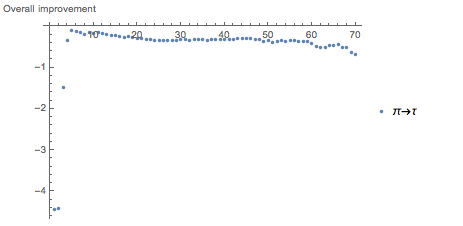where the least worsening, -0.04, is achieved at a complexity of 5. As you can see, the improvement stays below 0 the whole time, meaning that while more formulas may be shorter with τ (depending on the field), on average those length decreases are outweighed by the length increases in the formulas that are getting longer.

To make my point, at the end of this scientific investigation: I think we should be happy with our old friend π and not switch to τ.

I have two final observations. The first is that if we had already lived in a τ world, the conclusion would have been different, and we would have chosen to stick with τ. If our expressions were already in τ and we were investigating whether switching to π would make them simpler, our vector-based graph would look like this: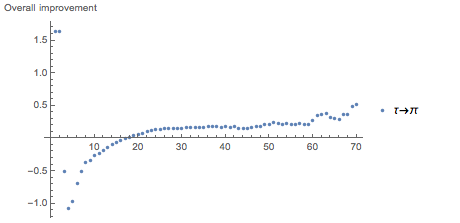That difference in behavior is because the vectors used to construct the graphs depend on the original complexities, and so change when the original changes.

This shows that for formulas that have a complexity greater than 2 (most of them do) and for which the complexity is not always greater than 18, the improvement in switching from τ to π would be negative again, suggesting that we should not accept the switch. Unfortunately for supporters of τ, we do not live in a τ world.

The second observation, which was brought to me by Michael Trott, is that 2/3 of the formulas shown in The Tau Manifesto (the green table at the beginning) don’t just have 2π in them, but the complex number 2πi. This suggests that maybe the question I was trying to answer is not the correct one. A better one could be this: would it make sense to have a new symbol τ for the complex number 2πi?

This new convention would require changing from πi to τ/2 as well, but that doesn’t affect the complexity of πi. In general, formulas having a πi term inside would either become simpler or preserve their complexity. To give you an idea, here’s a word cloud of formulas that would become simpler: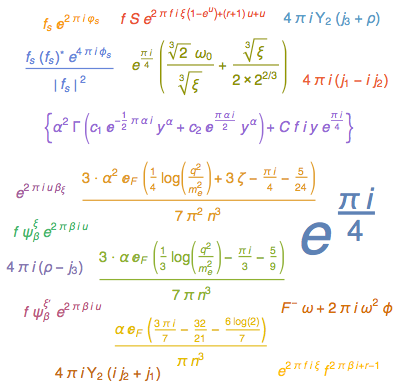Which, after substituting τ= 2πi, become these: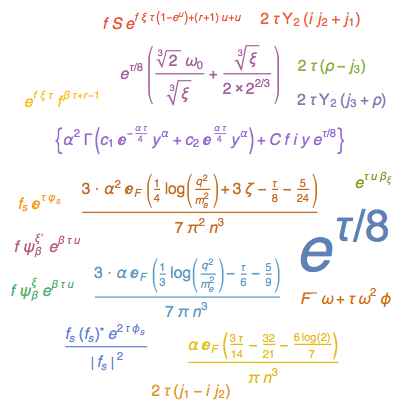You could argue that the percentage of improved formulas may not be high enough, and changing from 2πi to τ is not worth the effort. What evidence shows, however, is the opposite: of all formulas having a πi term, 75% would be simpler, and the remaining 25% would keep their original complexity—none would get worse. This is a strong point to make, and I am not in the position to do it, but I think the equality τ = 2πi looks more promising (and less historically disruptive) than τ = 2π.

Whatever your opinion on τ, I hope you have a lovely Tau Day. Please enjoy two pi(e)s today—imaginary or otherwise.